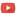KE8QZC | SFW | TSWSyllabus
WeBWork (online homework)

Homework
For Exam 1 (all due by 18 September 2023)
Homework 1: Review of functions
Homework 2: Limits (not limits as $x\rightarrow \pm\infty$)
Homework 3: Continuity and intermediate value theorem (only continuity part)

For Exam 2 (all due by 23 October)
Homework 4: Definition of differentiation and differentiation of polynomials
Homework 5: Nonpolynomial functions and the product and quotient rules

For Exam 3 (all due by 27 November)

Quizzes
For Exam 1
Quiz 1 (29 Aug 2023) [soln]: #1: Solve $x^2+3x+1=0$. #2: Solve $2^{x+1}=3^{3x-2}$.
Quiz 2 (31 Aug 2023) [soln]: Compute limits based on a graph (see pdf)
Quiz 3 (7 Sep 2023) [soln]: Compute $\displaystyle\lim_{t \rightarrow 2} \dfrac{t^2-t-2}{t^2+3t-10}$.

For Exam 2
Quiz 4 (12 Sep 2023) []: Use the intermediate value theorem to estimate a root of the polynomial $f(x)=x^7-5x^6+x^5-x^2+5x-1$. Your solution should contain at least two steps in the root-finding process. (note: $x=1$ is a root -- don't use that as an endpoint of any interval!)
Quiz 5 (19 Sep 2023) []: Let $f(t)=2t^2+3t$. Use the limit definition of the derivative to compute $\dfrac{\mathrm{d}f}{\mathrm{d}t}$.
Quiz 6 (22 Sep 2023) []: Compute $\dfrac{\mathrm{d}}{\mathrm{d}x} \Big[ x^7 - 5x^6 + x^5 - x^2 + 5x -1 \Big]$.

For Exam 3

Exams
Exam 1 (15 September)
Exam 2 (20 October)
Exam 3 (17 November)